How to Calculate Mean in Excel

The arithmetic means, mode and median are the base of any statistical analysis of data. We have already discussed how we can calculate MODE and MEDIAN in Excel. In this tutorial, we will learn how to calculate mean in excel.
Mean is nothing but the average of data. A given set of data is added and divided by total numbers. For example, mean of 2,4 and 8 will be (2+4+8)/3, which is 7.
In excel, we use the AVERAGE function to calculate the mean of data. The AVERAGE function does the same thing as mean. There is no MEAN function in excel since AVERAGE is there.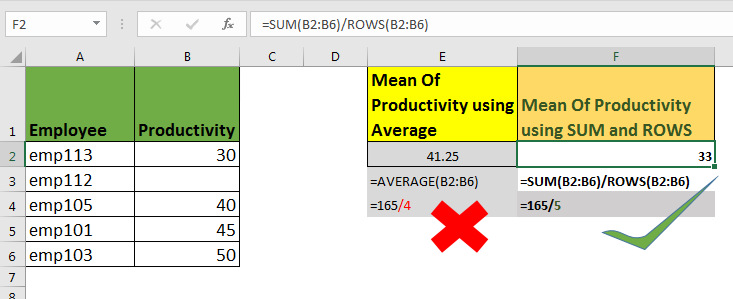Generic Mean Formula in Excel

=AVERAGE(number1,number2,...)

Number1,number2,... : These are the numbers of which you want to calculate mean in excel. This can be numbers, cell references and ranges.

The all below average formulas are valid.

=AVERAGE(1,4,8)
=AVERAGE(A1,A4,C8)
=AVERAGE(A2:B4,4,C12)

Excel Mean Examples
Here I have some data about weights of my colleagues in my company. Now, I want to calculate the mean in excel of this data.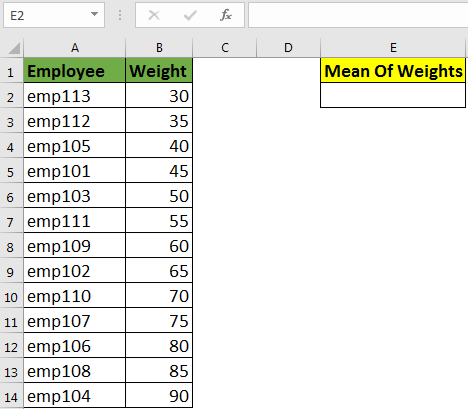Write this mean formula using AVERAGE function in excel:

=AVERAGE(B2:B14)

This will return the mean or say average of this data, which 60 kgs.

NOTE: any non numeric value is ignored except #DIV/0! And #N/A Error. If you have a #DIV/0! in set of numbers, AVERAGE function will return #DIV/0! error.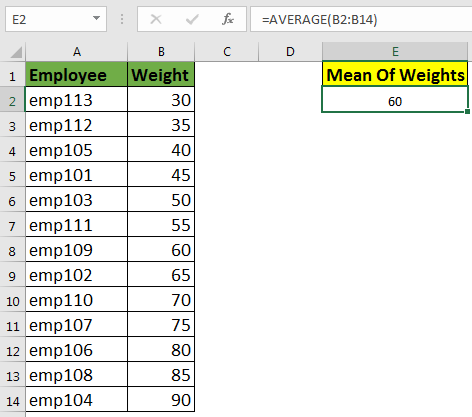Handling Blank Cells while Calculating MEAN in Excel
Above mean formula is equivalent to this formula:

=SUM(B2:B14)/COUNT(B2:B14)

It means if you have any blank cell or cell that contain non numeric value, it will be ignored and denominator will reduce. See the below image.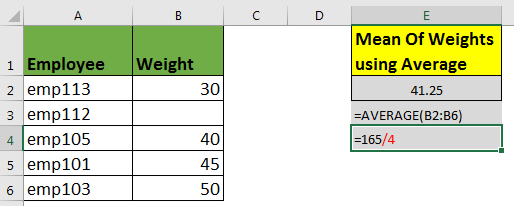See, we have, only 4 employees are taken in consideration. Which actually logical here, because no one can
weigh 0 Kgs on Earth.
But what if we are talking about productivity, that can be 0. In that case this analysis will be wrong.
To keep every record in consideration while calculating, either fill each non-numeric value with 0, or use below formula.

=SUM(B2:B6)/ROWS(B2:B6)How it Works

Here first SUM function adds all the non numeric value in the given range. Then ROWS returns the number of rows in the range. Eventually we divide the sum by number of rows to calculate the mean in excel.

Calculate MEAN using SUM and COUNTA Function.
Almost every data has some tags with it. For example, every weight and productivity is associated with an employee. So to calculate true mean, we can sum the number using SUM function and count the employees using COUNTA function. And then divide the SUM by COUNT of employee to calculate mean of productivity.

Formula to Calculate Mean of Data using SUM and COUNTA

=SUM(B2:B6)/COUNTA(A2:A6)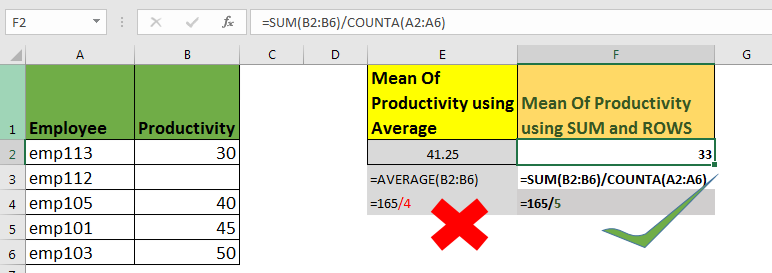So, yeah guys, these are the ways to calculate error free mean of a dataset. Feel free to ask question about any statical function in excel. And share you tricks to others and make others work easy.

Related Articles:

How to use the MODE function in Excel

How to use the MEDIAN function in Excel

Create Histograms in Excel 2016/2013/2010

Perform Regressions in excel

Popular Articles

50 Excel Shortcut to Increase Your Productivity : Get faster at your task. These 50 shortcuts will make you work even faster on Excel.

How to use the VLOOKUP Function in Excel : This is one of the most used and popular functions of excel that is used to lookup value from different ranges and sheets.

How to use the COUNTIF function in Excel : Count values with conditions using this amazing function. You don't need to filter your data to count specific values. Countif function is essential to prepare your dashboard.

How to use the SUMIF Function in Excel : This is another dashboard essential function. This helps you sum up values on specific conditions.

Terms and Conditions of use

The applications/code on this site are distributed as is and without warranties or liability. In no event shall the owner of the copyrights, or the authors of the applications/code be liable for any loss of profit, any problems or any damage resulting from the use or evaluation of the applications/code.